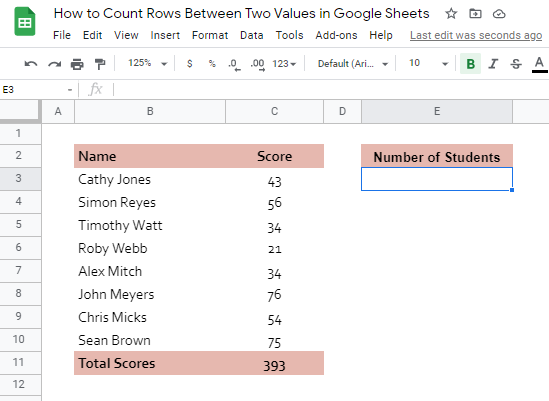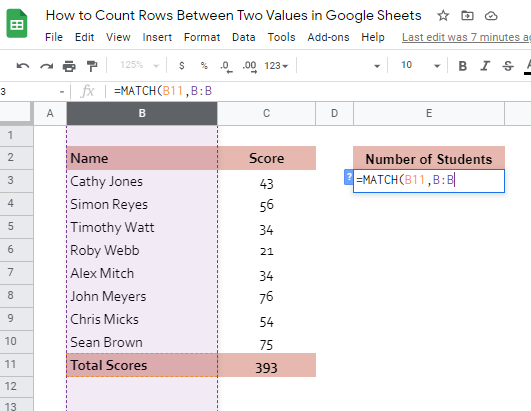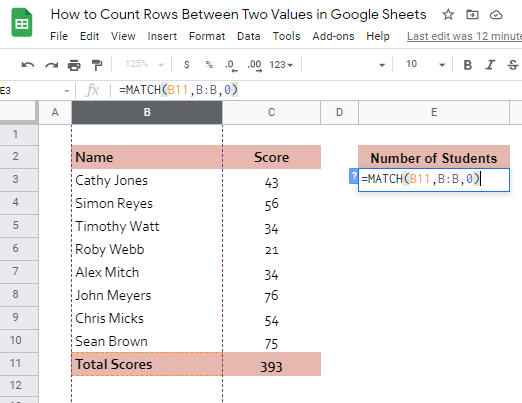# How to Count Rows Between Two Values in Google Sheets

Knowing how to count rows between two values in Google Sheets is useful if you want to find out how far one value is from another in terms of the number of rows.

Meaning, regardless of whether there are values in between or not, this article would teach you how to generate or write a formula to count the rows between two specific values.

There is no specific function that we can use to be able to achieve this task. What we will use, instead, is a combination of function and formula. Please see the rules below before we continue further:

• The MATCH function is the main function of this process. It returns the relative position of an item within the specified range. Feel free to visit this MATCH function article for a more detailed explanation and samples.
• If multiple instances of search value appear in the column that we’re searching into, the MATCH function returns the row number of the first instance. This will possibly cause issues in the process of counting the rows between cell values.

Let’s take an example.

Please take a look at the table below:Jenny, an inventory clerk to a mini fruit store, is asked to prepare a report that shows the total number of days each fruit item has been sold for quarter three.

While there may be other ways to do it, she used the very simple trick she knows. Jenny thought that if she’d count the rows between the fruit items, then that could be the values she’s looking for.

Let’s have another real-life sample!

Teacher Brent is keeping his records organized in Google Sheets. One of his records is scoresheets for every quiz he provides to his students. Please see one of the samples below:To be able to always make him aware of the number of students he has, Brent tends to create an identifier on each of his scoresheets. Check how he was able to identify the number of students above. Note that this number auto-adjusts as soon as he adds or removes students’ names. How convenient is that!

Brent simply counts the rows between the text ‘Name’ and ‘Total Scores’. Easy right?

Watch out for a more advanced tutorial and examples on how you can count rows between two values in the coming weeks. Be sure to subscribe to be notified.

Awesome! Let’s begin getting to know more about the steps and processes in counting rows between two values in Google Sheets.

## The Anatomy of the MATCH Function used for counting row between values

So the syntax (the way we write) the MATCH function is as follows:

`=MATCH(search_key, range, [search_type])`

Let’s dissect this thing and understand what each of these terms means:

• = the equal sign is just how we start any function in Google Sheets. It is how Google Sheets understand that we are asking it to either do a computation or use a function.
• MATCH() this is our main function.
• search_key is the value we will search for within the range.
• range the one-dimensional array to be searched for the search_key.
• search_type is the manner in which to search [optional].

## A Real Example of Counting Rows Between Two Values

Take a look at our fruit inventory example below to see how the MATCH function is used in obtaining the number of rows between two specific values.In the example above, our goal is to find the distance between the word ‘Apples’ and ‘Oranges’ in terms of rows.

Take note of how many instances MATCH function were used in the formula. The first one is used to be able to get the relative position of the word ‘Orange’, which is 9 (Row 9).

The second match function was used to get the relative position of the word ‘Apples’, which is 3 (Row 3). These values, 9 and 3, are vital in getting the number of rows between the words ‘Apples’ and ‘Oranges’. How?

It’s like 9-3-1, which is 5. So basically, the formula should be:

First MATCH function Second MATCH function – 1Amazing right? Who would have thought that a combination of arithmetic operation and two MATCH functions would come in handy? 🙂

Please know that the 1 on the last part of the formula is constant on this kind of scenario. However, it could change depending on your goal.

See our second example below:

What if we want to find out the combined number of days of Apples and Oranges?Since we will be calculating the distance between the text value ‘Apples’ and ‘Pears’ now, the first argument of both our MATCH functions will be changed to ‘Pears’ and ‘Apples’ for the first function and second function, respectively.Notice that we are now subtracting 2, instead of 1, from the difference of two MATCH functions. It is because of the extra rows that shouldn’t be included in the count:That’s how we are able to come up with the value 13, which is the total number of days the Apples and Oranges were sold.You may make a copy of the spreadsheet using the link I have attached below.

## How to Count Rows Between Two Values in Google Sheets

1. Click on any cell to make it the active cell. For this guide, I will be selecting E3, where I want to show my result.1. Next, type the equal sign ‘=‘ to begin the function and then followed by the name of the function, which is our ‘match‘ (or ‘MATCH‘, not case sensitive).

1. Type open parenthesis ‘(‘ or simply hit Tab key to let you use that function.1. Now the fun part! Let’s give our first MATCH function its first argument, which is the text value to look for. You may pass constant data by typing the exact text value. For this example, I’ll be using the cell address of the text value ‘Total Scores’. Type in B11.1. To let the Google sheet know that we’re done typing our first argument, we should now type in the delimiter or the character that separates each argument on a function. In this case, type comma,’ followed by the next argument, which is the range to be searched for the text value ‘Total Scores’. Type in B:B.1. Type in comma (,) followed by the last argument, which is 0. 0 since we are looking for the exact match in column B of the word ‘Total Scores’.1. Finally, end the first function by typing in close parenthesis )’.1. To let the Google Sheet know that we are subtracting a value from our first MATCH function, type in hyphen’ followed by our second MATCH function.1. Pass the first argument of our second MATCH function, which is the cell address of the text value ‘Name’. Type in B2 followed by the delimiter comma ,’.1. Provide the second argument, which is the column B or simply type in B:B, followed by comma,’ and the third argument, which is 0.1. Close the second MATCH function by typing in the closing parenthesis )’.1. Now, subtract 1 by continuing typing in hyphen’ and 1.1. Finally, hit Enter or your Tab key. Cell E3 should now show you the distance between the text values ‘Name’ and ‘Total Scores’. For Brent, on his scoresheet, it should identify how many students he has.That’s pretty much it. You can now count rows between two values in Google Sheets together with the other numerous Google Sheets formulas to create even more powerful formulas that can make your life much easier.Our goal this year is to create lots of rich, bite-sized tutorials for Google Sheets users like you. If you liked this one, you'll love what we are working on! Readers receive ✨ early access ✨ to new content.

##### You May Also Like## How to Extract Date From Timestamp in Google Sheets

To extract date from timestamp in Google Sheets is useful if you have DateTime values in your sheet,…## How To Use IMCOS Function in Google Sheets

The IMCOS function in Google Sheets is useful when you would like to retrieve the cosine of a…## How to Use DSUM Function in Google Sheets

The DSUM function in the Google Sheets formula is very similar to the SUM function, but with one…## How to Link Data from a Different Tab in Google Sheets

When working with Google Sheets, it might be helpful to link data from a different tab in your…